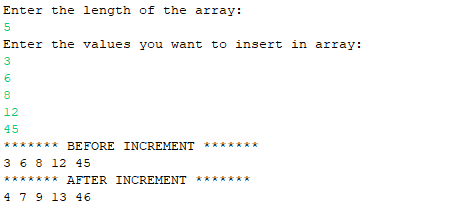# Java Program to Increment All Element of an Array by One

• Last Updated : 11 Nov, 2020

Given the array, the task is to increment each element of the array by 1. Complete traversal is required for incrementing all the elements of an array. An optimized way to complete a given task is having time complexity as O(N).

Examples:

Attention reader! Don’t stop learning now. Get hold of all the important Java Foundation and Collections concepts with the Fundamentals of Java and Java Collections Course at a student-friendly price and become industry ready. To complete your preparation from learning a language to DS Algo and many more,  please refer Complete Interview Preparation Course.

```Input : arr1[] = {50, 25, 32, 12, 6, 10, 100, 150}
Output: arr1[] = {51, 25, 33, 13, 7, 11, 101, 151}

Input : arr2[] = {3, 6, 8, 12, 45}
Output: arr2[] = {4, 7, 9, 13, 46}
```

1. with pre-defined values:

First, Initializing the array arr[] with pre-defined values, and after that, the length of the array should be calculated. Then use a loop, to perform the operation that is to increment the values one by one with the help of for loop. After that, the resultant of the above operation will get stored into the array and then the output will be shown with the help of for loop one by one.

## Java

 `// Java Program to Increment All``// Element of an Array by One``public` `class` `Increment {``    ``public` `static` `void` `main(String[] args)``    ``{``        ``// assigning values in array``        ``int``[] arr = { ``50``, ``25``, ``32``, ``12``, ``6``, ``10``, ``100``, ``150` `};``       ` `        ``// finding the length of the array``        ``// and assigning it to the variable n``        ``int` `n = arr.length;`` ` `        ``System.out.println(``            ``"******* BEFORE INCREMENT *******"``);``       ` `        ``// printing the elements of array``        ``// before performing operation``        ``for` `(``int` `i = ``0``; i < n; i++) {``            ``System.out.print(arr[i]);``            ``System.out.print(``" "``);``        ``}`` ` `        ``// incrementing the values of array``        ``// by 1 with the help of loop``        ``for` `(``int` `i = ``0``; i < n; i++) {``            ``arr[i] = arr[i] + ``1``;``        ``}`` ` `        ``System.out.println(``" "``);``       ` `        ``// elements of the array after increment``        ``System.out.println(``            ``"******* AFTER INCREMENT *******"``);``       ` `        ``// printing the elements of array after operation``        ``for` `(``int` `i = ``0``; i < n; i++) {``            ``System.out.print(arr[i]);``            ``System.out.print(``" "``);``        ``}``    ``}``}`
Output
```******* BEFORE INCREMENT *******
50 25 32 12 6 10 100 150
******* AFTER INCREMENT *******
51 26 33 13 7 11 101 151
```

Time Complexity: O(N), Where N is the size of an array

2. with user-defined values:

Initializing the array arr[] with user-defined values i.e. the values of the array will be given by the user, and after that, the length of the array should be calculated. Then use a loop, to perform the operation that is to increment the values one by one with the help of for loop. After that, the resultant of the above operation will get stored into the array and then the output will be shown with the help of for loop one by one.

## Java

 `// Java Program to Increment All``// Element of an Array by One``import` `java.util.Scanner;`` ` `public` `class` `Increment2 {`` ` `    ``public` `static` `void` `main(String[] args)``    ``{``        ``System.out.println(``            ``"Enter the length of the array: "``);``       ` `        ``// The object of scanner class is created``        ``// in order to take the input from user``        ``Scanner sc = ``new` `Scanner(System.in);``       ` `        ``int` `n = sc.nextInt();``       ` `        ``// Initializing the array of size that``        ``// is given by the user``        ``int``[] arr = ``new` `int``[n];``       ` `        ``// Calculating the length of the array``        ``int` `l = arr.length;`` ` `        ``System.out.println(``            ``"Enter the values you want to insert in array: "``);``       ` `        ``// Taking the values from user``        ``for` `(``int` `i = ``0``; i < l; i++) {``            ``arr[i] = sc.nextInt();``        ``}`` ` `        ``System.out.println(``            ``"******* BEFORE INCREMENT *******"``);``       ` `        ``// The elements of array before increment``        ``for` `(``int` `i = ``0``; i < l; i++) {``            ``System.out.print(arr[i]);``            ``System.out.print(``" "``);``        ``}`` ` `        ``// Incrementing the values of the array by 1``        ``for` `(``int` `i = ``0``; i < l; i++) {``            ``arr[i] = arr[i] + ``1``;``        ``}`` ` `        ``System.out.println(``" "``);``        ``System.out.println(``            ``"******* AFTER INCREMENT *******"``);``        ``// Elements of array after increment``        ``for` `(``int` `i = ``0``; i < l; i++) {``            ``System.out.print(arr[i]);``            ``System.out.print(``" "``);``        ``}``    ``}``}`

Output:Time Complexity: O(N), where N is the size of an array

My Personal Notes arrow_drop_up